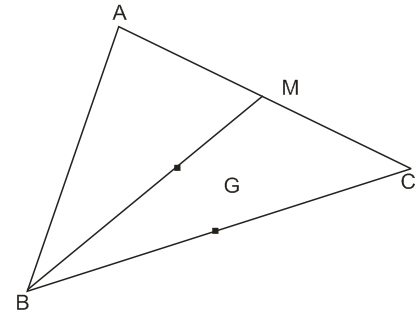Q

# Stuck here, help me understand: - Three Dimensional Geometry - JEE Main-6

Let  be the

vertices of a triangle and M be the midpoint of AC. If G divides

BM in the ratio , 2:1, then  ( O being the origin)

is equal to :

• Option 1)

• Option 2)

• Option 3)

• Option 4)

ViewsGiven that M is mid point of AC and G divides BM in the ratio 2:1

G is centroid of ABC

So, correct option is (2)

Option 1)

Option 2)

Option 3)

Option 4)

Exams
Articles
Questions